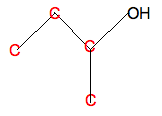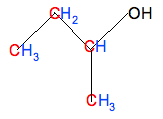Chemguide: Support for CIE A level Chemistry ``` ``` Learning outcome 13 13.1: Formulae, functional groups and the naming of organic compounds ``` ``` Statement 13.1.6 This statement is about working out the molecular or empirical formula of an organic compound from its structure. Before you go on, you should find and read the statement in your copy of the syllabus. ``` ``` Working out the molecular formula If you are given the structural formula of, say, ethanol as CH3CH2OH, it is easy to add up the various atoms to give the molecular formula of C2H6O. If you are given it as a displayed formula, you just have to be careful to count all the atoms. It isn't difficult - you just have to be careful. It is definitely more difficult to do this from a skeletal formula, though. If I was doing this in an exam, I would write in all the missing atoms on top of the structure on the question paper. So, starting with a skeletal formula:Write in all the carbon atoms:Don't forget that there isn't a carbon atom at the end of a line with something already attached - in this case, an -OH group. And then add enough hydrogens so that each carbon is making four bonds:. . . giving the molecular formula C4H10O. Be careful, though, and take your time. It is very easy to miss something - for example, in this case, forgetting to count the hydrogen in the -OH group! Working out the empirical formula The empirical formula simply tells you the simplest ratio of the atoms present, and I don't really understand why CIE are asking you to do this! More usually you would have to use the empirical formula in order to find the molecular formula. I can't think of any reason why you should ever want to find an empirical formula for its own sake. Anyway . . . If your molecular formula turned out to be C2H4O2, then the simplest ratio is 1C:2H:1O. The empirical formula is therefore CH2O. If your molecular formula turned out to be C6H6, then the simplest ratio is 1C:1H. The empirical formula is therefore CH. Quite often, the empirical formula is the same as the molecular formula. For example, in the molecular formula C2H6O, you can't simplify the ratio any more. So the empirical formula is also C2H6O. ``` ``` Go to the Section 13 Menu . . . To return to the list of learning outcomes in Section 13 Go to the CIE Main Menu . . . To return to the list of all the CIE sections Go to Chemguide Main Menu . . . This will take you to the main part of Chemguide. © Jim Clark 2020